# Civil Engineering - UPSC Civil Service Exam Questions

6.

The maximum bending moment at the left quarter point of a simple beam due to crossing of UDL of length shorter than the span in the direction left to right, would occur after the load had just crossed the section by

 A. one-fourth of its length B. half of its length C. three-fourth of its length D. its full length

Explanation:

No answer description available for this question. Let us discuss.

7.

The formulation for BOD assimilation in a stream should include

 A. BOD rate constant B. Sedimentation of organic matter C. BOD rate constant and sedimentation of organic matter D. Pathogenic bacterial decay coefficient

Explanation:

No answer description available for this question. Let us discuss.

8.

A truss is shown in the given figure. The cross-sectional area of each member is 'A' and the modulus of elasticity of the material is E. The strain energy in the member XY is given by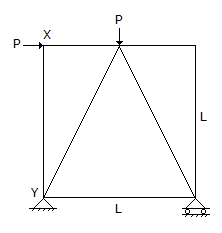A. P2L/2AE B. P2L/QAE C. P2L/3AE D. zero

Explanation:

No answer description available for this question. Let us discuss.

9.

For the simply supported beam shown in the given figure, at what distance from the support A, is the shear force zero?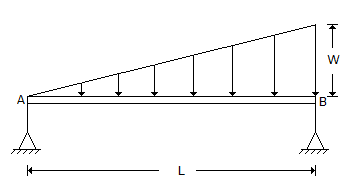A.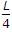B.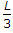C.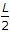D.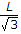Explanation:

No answer description available for this question. Let us discuss.

10.

The elements that are normally subjected to combined bending and axial forces are

 A. struts in reinforced concrete members B. the members in long span bridges C. columns in framed structures D. space truss members